# High School Physics : Understanding Newton's Third Law

## Example Questions

### Example Question #2 : Newton's Laws

If a tennis ball hits a racket with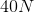of force, what is the force of the racket on the ball?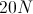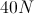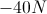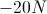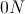Explanation:

Newton's third law states that every force has an equal and opposite force.

That means that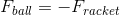. Since the force of the ball equals, the force of the racket must be equal and opposite.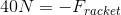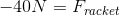.

Remember, this force will be negative as it is equal and OPPOSITE. That means it is moving in the opposite direction.

### Example Question #2 : Understanding Newton's Third Law

A bat hits a baseball with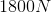of force. How much force does the baseball exert on the bat?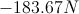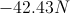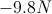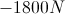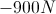Explanation:

Newton's third law states that when object A exerts a force on object B, object B exerts a force equal in magnitude but opposite in direction on object A.

That means that if the force of the bat on the ball is, then the ball on the bat must be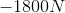.

### Example Question #11 : Newton's Laws

A hammer strikes a nail withnewtons of force. How much force does the nail exert on the hammer?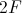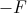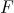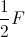Explanation:

Newton's third law states that for every force, there is an equal and opposite force. The force of the hammer on the nail will be equal in magnitude, but opposite in direction, to the force of the nail on the hammer.

Mathematically, that would mean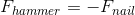.

The nail would exert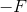force on the hammer.

### Example Question #4 : Understanding Newton's Third Law

Two skaters push off of each other in the middle of an ice rink. If one skater has a mass of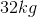and an acceleration of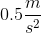, what is the mass of the other skater if her acceleration is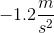?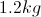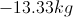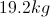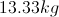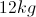Explanation:

For this problem, we'll use Newton's third law, which states that for every force there will be another force equal in magnitude, but opposite in direction.

This means that the force of the first skater on the second will be equal in magnitude, but opposite in direction: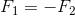Use Newton's second law to expand this equation.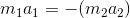We are given the acceleration of each skater and the mass of the first. Using these values, we can solve for the mass of the second.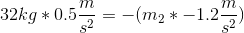Notice that the acceleration of the second skater is negative. Since she is moving in the opposite direction of the first skater, one acceleration will be positive while the other will be negative as acceleration is a vector. From here, we need to isolate the mass of the second skater.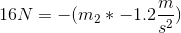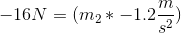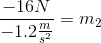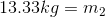### Example Question #5 : Understanding Newton's Third Law

Two skaters push off of each other in the middle of an ice rink. If one skater has a mass ofand an acceleration of, what is the acceleration of the other skater if her mass is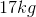?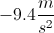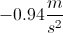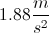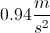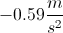Explanation:

For this problem, we'll use Newton's third law, which states that for every force there will be another force equal in magnitude, but opposite in direction.

This means that the force of the first skater on the second will be equal in magnitude, but opposite in direction:Use Newton's second law to expand this equation.We are given the mass of each skater and the acceleration of the first. Using these values, we can solve for the acceleration of the second.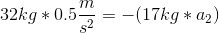From here, we need to isolate the acceleration of the second skater.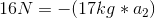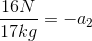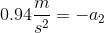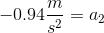Notice that the acceleration of the second skater is negative. Since she is moving in the opposite direction of the first skater, one acceleration will be positive while the other will be negative as acceleration is a vector.

### Example Question #11 : Newton's Laws

A rock strikes a window with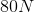of force. How much force does that window exert on the rock?We need to know how long the two were in contact to solve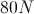We need to know the mass of the rock to solve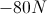Explanation:

Newton's third law states that when one body exerts a force on another body, the second body exerts a force equal in magnitude, but opposite in direction, on the first body.

Mathematically, this process can be written as:Since the rock exertsof force on the window, then the window must exert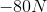of force on the rock.

### Example Question #1 : Understanding Newton's Third Law

A boy falls out of a tree and hits the ground with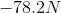of force. How much force does the ground exert on the boy?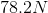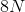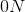We must know the mass of the boy to solveExplanation:

Newton's third law states that when one object exerts a force on another object, that second object exerts a force of equal magnitude, but opposite in direction on the first.

That means that:Using the value from the question, we can find the force of the ground on the boy.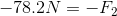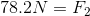### Example Question #8 : Understanding Newton's Third Law

A hunter fires a rifle. The rifle kicks back with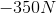of force. How much force does the hunter's shoulder exert on the rifle during kick back?We don't have enough information to solve.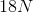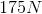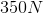Explanation:

If the rifle exerts a force ofon the hunter's shoulder, then the hunter's shoulder will exertof force on the rifle.

This is because of Newton's third law, which states that when one object exerts a force upon another object, the second object exerts a force equal in magnitude but opposite in direction to the first force. Mathematically that means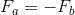. Since our first force was, the second force will be.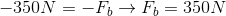### Example Question #1 : Understanding Newton's Third Law

Which of Newton's laws explains why it is easy for you to lift a 1L jug of milk from the fridge, but impossible to lift a 1000L jug?

None of these

Newton's first law

Newton's third law

More than one of these

Newton's second law

The answer is more than one. The two laws that come into play are Newton's first and second laws. Newton's first law is best known as the law of inertia. It states that an object in motion will stay in motion and an object at rest will stay at rest unless acted on by an outside force. Newton's second law relates the acceleration of an object to the mass and the forces acting on it with the equation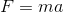. An object won't move or stop moving unless the forces acting on it are imbalanced. In the case of the milk jug, it remained at rest until an outside force, your hand, acted upon it, demonstrating Newton's first law. The second law is applicable because the amount of acceleration of the two milk jugs is inversely related to their mass. It takes a much stronger force to move the 1000L milk jug than it does the 1L milk jug. The acceleration is also much smaller for the two objects when the same force is applied because one weighs so much more than the other. You can visualize this by rearranging the second law equation: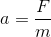. It is also beneficial to think in terms of two objects. Which is will accelerate more when you try and move it with the same force, a tennis ball or an elephant?